Previous: Formulas for the Efficiency Up: Formulas for the Efficiency Next: Pump Efficiency
This is an automatically generated documentation by LaTeX2HTML utility. In case of any issue, please, contact us at info@cfdsupport.com.

## Hydro Turbine Efficiency

The water turbine efficiency can be evaluated using following efficiency formula: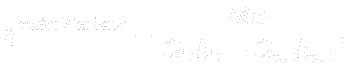where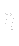denotes the efficiency,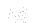is the torque,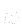is the angular velocity,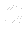is the volume flow rate andis the specific enthalpy which can be evaluated as follows: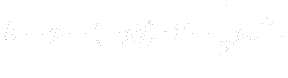Subscript in means averaged quantities at the inlet, whereas subscript out denotes averaged quantities at the outlet. The evaluation of turbine efficiency for different patches is also possible.